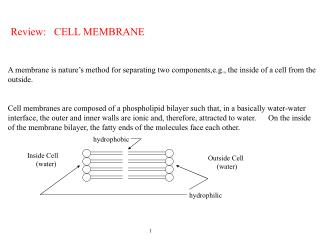DownloadDownload PresentationReview: CELL MEMBRANE

# Review: CELL MEMBRANE

Download Presentation## Review: CELL MEMBRANE

- - - - - - - - - - - - - - - - - - - - - - - - - - - E N D - - - - - - - - - - - - - - - - - - - - - - - - - - -
##### Presentation Transcript

1. Review: CELL MEMBRANE A membrane is nature’smethod for separating two components,e.g., the inside of a cell from the outside. Cell membranes are composed of a phospholipid bilayer such that, in a basically water-water interface, the outer and inner walls are ionic and, therefore, attracted to water. On the inside of the membrane bilayer, the fatty ends of the molecules face each other. hydrophobic Inside Cell (water) Outside Cell (water) hydrophilic 1

2. But ‘things’ must pass into and out of cells, i.e., through membranes This is accomplished by (at least) 3 different methods: (i) a substance dissolving (ionizing) in the phospholipid and diffusing through (ii) passing through water-filled pores (iii) by means of carriers The last two imply that things are imbedded in the membrane allowing passage of some ions and water (easily). 2

3. Forces on Particles For a substance to be in equilibrium across a membrane, the work required to bring a substance to points just inside or just outside the membrane must be equal. Definitions: One volt is the work needed to bring a unit positive charge from infinity to a particular point. (particle of valence #z) WE = z F V where units are F - coulombs/ mole V - joules/coulomb WE - Elec. work Concentration is the work needed to bring a substance from 1 mole/liter to the concentration in the cell. WC = R T ln[S] where units are R - gas constant T - degrees Kelvin [S] - conc. of S WC - chem. work

4. Semi-Permeable Membranes Diffusion after time No Net Flow Net Flow Charged particles set up electric field E = F / q (Newtons/Coulomb) Potential Gradient High energy particles cross the membrane

5. Semi-Permeable Membranes Large Neg. Ions Large Pos. Ions Large Pos. Ions Large Neg. Ions Charge neutral on both sides of membrane E- D+ D+ after time D- D- E+ - - D - diffusion E - Elec. field + + D + E = D + E (currents in dynamic equilibrium)

6. Osmotic Balance Semi-permeable Membrane Diffusion will drive water to equalize concentrations inside and outside the cell Fick’s Law of Diffusion dS/dt = D (C1 - C2) where S- solute Ci - concentration in ith compartment P (solute) S (solute) Water D - diffusion constant For water to reach equilibrium [S]i + [P]i = [S]o Note: [P]o = 0 If [P]i is not zero, water will be driven osmotically into the cell so that it will swell perhaps to the point of membrane failure (burst).

7. Cell Model (in equilibrium) Assume [K+]o = 5mM, [Na+]o = 120 mM, [Cl-]i = 5mM, [A- ]i = 108mM where A- is a membrane-impermeable ion. For electrical neutrality outside, [Cl-]o = 125mM From Donnan equilibrium, [K+]i = 125 mM Inside 12 mM Na+ 125 mM K+ 5 mM Cl- 108 mM A- Outside Na+ 120 mM K+ 5 mM Cl- 125 mM Total osmolarity=250 mOsm Total osmolarity=250 mOsm membrane From the Nernst Equation for either Cl- or K+, the membrane potential is Vm = (R T/zClF) ln{[ Cl-]o/[Cl-]i} = (R T/zKF) ln{[ K+]o/[K+]i} (for Cl ) = (-58 mV) log{ 125 mM/5mM} = (-58) 1.398 = -81 mV However, real cells are NOT IN EQUILIBRIUM so they must expend metabolic energy to maintain the status quo, I.e., this model needs modification.

8. Nernst Equation (for one substance in equilibrium across membrane) Where subscript i - inside cell o - outside cell Zs F Vi + R T ln[S]i = Zs F Vo + R T ln[S]o If Vm = Vi - Vo, Vm = (RT)/ (ZsF) ln([S]o/ [S]i) Nernst Equation If there are two substances, the Nernst Eq’n readily leads to the Gibbs-Donnan Eq’n. Vm = (RT/Z1F) ln( [S1]o/[S1]i) = (RT/Z2F) ln( [S2]o/[S2]i) So (see blackboard for equil. of 2 substances across membrane)

9. We find (under physiological conditions) using the Nernst equation that the equilibrium potential for K ~ -76mv and for Na ~ +55 mv. The measured membrane potential is ~ -70 mv so we conclude that in ‘real life’, the Na ion in particular is not in equilibrium, i.e., the membrane is not completely impermeable to the Na ion. The membrane potential is described well by the Goldman Equation which assumes a constant E-field across the membrane and assumes non-equilibrium membrane potential for the three ions Na+, K+, Cl- The Goldman Equation is Vm = (R T/F) ln[{a [K]o + b[Na]o + c[Cl] i}/ {a[K]i + b[Na]i + c[Cl]o} ] where a, b, c are the permeabilities of the membrane to K, Na, Cl respectively

10. Electrolytes and Potentials Across Membranes External Internal Na+ 100 mM/l Na+ 10 No diffusion potential Cl- 100 mM/l Cl- 10 H2O Small pores implies NO IONS PASS

11. Initially electrically neutral External Internal External Internal + - 10 mV Na+ 50 mM/l Na+ 100 Na+ 64 mM/l Na+ 86 R+ 50 mM/l R+ 50 mM/l Cl- 100 mM/l Cl- 100 Cl- 114 mM/l Cl- 86 mM/l 200 mM/l Osmotic Concentration 228 mM/l 172 mM/l Osmotic Concentration E= (RT/F) ln (Na int / Na ext) Large pores implies MODERATE SIZE IONS PASS